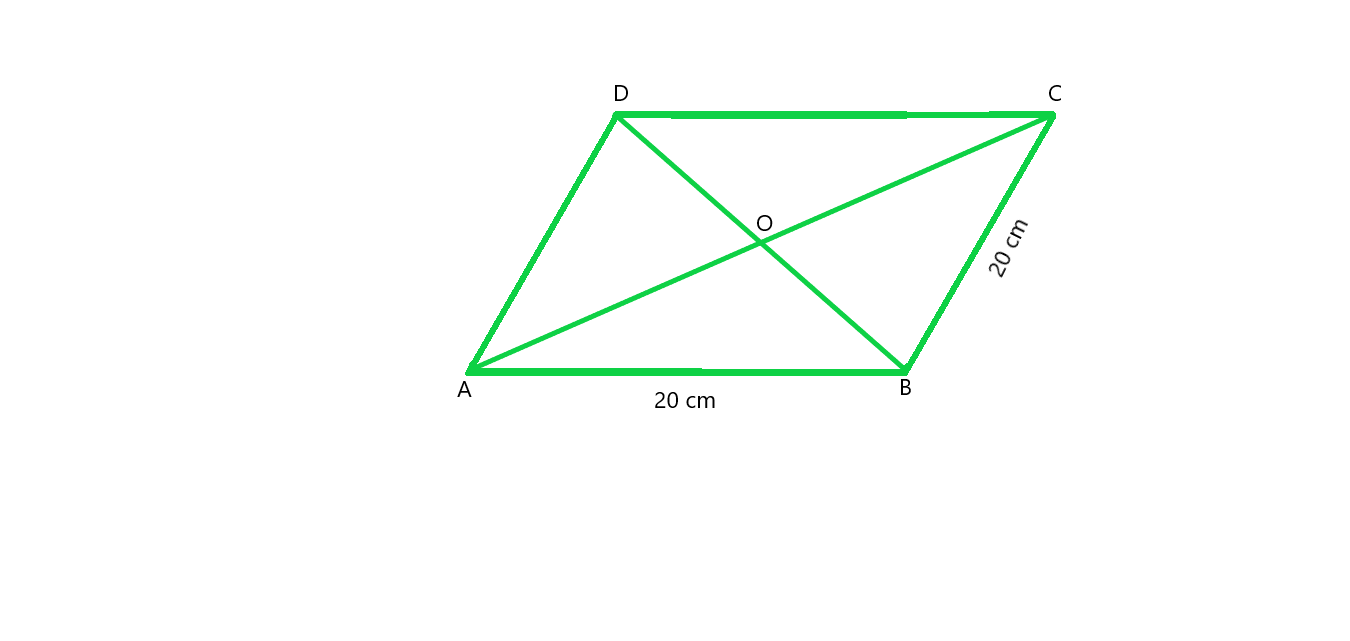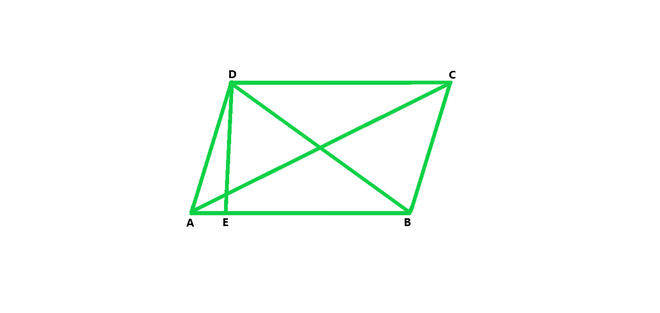GFG App
Open AppBrowser
Continue

# Class 8 RD Sharma Solutions – Chapter 20 Area Of Trapezium And Polygon- Exercise 20.1 | Set 2

### Question 12. The floor of a building consists of 3000 tiles which are rhombus shaped and each of its diagonals are 45 cm and 30 cm in length. Find the total cost of polishing the floor, if the cost per m2 is Rs. 4.

Solution:

As we know that,

Area of rhombus = 1/2 × d1 × d2

Area of rhombus = 1/2 × 45 × 30

Area of rhombus = 1350/2 = 675

Hence, Area of rhombus = 675 cm2

Area of one tile = 675 cm2                                (Given)

Now, Area of 3000 tiles = 675× 3000 = 2025000 cm2

Area of tiles in m2 = 2025000/10000 = 202.5 m2

Hence, Total cost for polishing the floor = 202.5× 4 = Rs 810

### Question 13. A rectangular grassy plot is 112 m long and 78 m broad. It has gravel path 2.5 m wide all around it on the side. Find the area of the path and the cost of constructing it at Rs. 4.50 per square meter.

Solution:

As we know that,

Outer area of rectangle = length × breadth

Outer area of rectangle = 112 × 78 = 8736 m2

Width of path = 2.5 m                                          (Given)

Length of inner rectangle = 112 – (2.5 + 2.5) = 107 m

Breadth of inner rectangle = 78 – (2.5 + 2.5) = 73 m

Inner area of rectangle = length × breadth

Inner area of rectangle = 107 × 73 = 7811 m2

Now we will calculate Area of path,

Area of path = Outer area of rectangle – Inner area of rectangle

Area of path = 8736 – 7811 = 925 m2

Cost of construction for 1 m^2 = Rs 4.50                                     (Given)

Hence, Cost of construction for 925 m2 = 925 × 4.50 = Rs 4162.5

### Question 14. Find the area of a rhombus, each side of which measures 20 cm and one of whose diagonals is 24 cm.

Solution:Given that,

Length of side of rhombus = 20 cm,

Length of one diagonal = 24 cm.

In ΔAOB,

Using Pythagoras theorem : AB2 = OA2 + OB2

202 = 122 + OB2

OB2 = 202 – 122

OB2 = 400 – 144

OB2 = 256

OB = 16

So, the length of the other diameter = 16 × 2 = 32 cm

As we know that Area of rhombus = 1/2 × d1 × d2

Area of rhombus = 1/2 × 24 × 32

Area of rhombus = 384 cm2

### Question 15. The length of a side of a square field is 4 m. What will be the altitude of the rhombus, if the area of the rhombus is equal to the square field and one of its diagonal is 2 m?

Solution:

Given that,

Length of a side of a square = 4 m,

Area of square = (side)2,

Area of square = 4 × 4 = 16 m2

As we know that,

Area of square = Area of rhombus

Therefore, Area of rhombus = 16 m2

Area of rhombus = 1/2 × d1 × d2

16 = 1/2 × 2 × d2

16 = d2

The diagonal of rhombus = 16 m                              (Given)In ΔAOB,

Using Pythagoras theorem

(AB)2 = (OA)2 + (OB)2

AB2 = 82 + 12

AB2 = 65

AB = √65

As we know that rhombus is a parallelogram, therefore area of parallelogram = base × altitude

Area of parallelogram = AB × DE

16 = √65 × DE

DE = 16/√65

Hence, Altitude of Rhombus = 16/√65 cm.

### Question 16. Find the area of the field in the form of a rhombus, if the length of each side be 14 cm and the altitude be 16 cm.

Solution:

Given that,

Side of rhombus = 14 cm,

Altitude of rhombus = 16 cm

As we know that rhombus is a parallelogram, therefore

Area of parallelogram = base × altitude

Area of parallelogram = 14 × 16 = 224 cm2

### Question 17. The cost of fencing a square field at 60 paise per meter is Rs. 1200. Find the cost of reaping the field at the rate of 50 paise per 100 sq. meters.

Solution:

As we know that, Perimeter of square field = Cost of fencing / rate of fencing

Perimeter of square field = 1200/0.6 = 2000

Hence, Perimeter of square field = 2000 m

As we know that Perimeter of square = 4 × side

Side of square = Perimeter / 4 = 2000/4 = 500

So, the Side of square = 500 m

As we know that, Area of square = side2

Area of square = 500 × 500 = 250000 m2

Cost of reaping = (250000 × 0.5) / 100 = 1250

Hence, Cost of reaping the field is Rs 1250

### Question 18. In exchange of a square plot one of whose sides is 84 m, a man wants to buy a rectangular plot 144 m long and of the same area as of the square plot. Find the width of the rectangular plot.

Solution:

As we know that,

Area of square = side2

Area of square = 84 × 84 = 7056

Since, Area of square = Area of rectangle

7056 = 144 × width

Width = 7056/144 = 49

Hence, Width of rectangle = 49 m

### Question 19. The area of a rhombus is 84 m2. If its perimeter is 40 m, then find its altitude.

Solution:

Given that,

Area of rhombus = 84 m2,

Perimeter = 40 m.

As we know that,

Perimeter of rhombus = 4 × side

Hence, Side of rhombus = Perimeter / 4 = 40/4 = 10

Side of rhombus = 10 m

Since rhombus is a parallelogram, therefore Area of parallelogram = base × altitude

84 = 10 × altitude

Altitude = 84/10 = 8.4

Hence, the Altitude of rhombus = 8.4 m

### Question 20. A garden is in the form of a rhombus whose side is 30 meters and the corresponding altitude is 16 m. Find the cost of levelling the garden at the rate of Rs. 2 per m2.

Solution:

Given that,

Side of rhombus = 30 m,

Altitude of rhombus = 16 m.

As we know that, rhombus is a parallelogram, therefore Area of parallelogram = base × altitude

Area of parallelogram = 30 × 16 = 480 m2

Cost of levelling the garden = area × rate

Cost of levelling the garden = 480 × 2 = 960

Hence, the Cost of levelling the garden is Rs 960

### Question 21. A field in the form of a rhombus has each side of length 64 m and altitude 16 m. What is the side of a square field which has the same area as that of a rhombus?

Solution:

Given that,

Side of rhombus = 64 m,

Altitude of rhombus = 16 m,

As we know that rhombus is a parallelogram, hence Area of parallelogram = base × altitude

Area of parallelogram = 64 × 16 = 1024 m2

Since Area of rhombus = Area of square

Therefore, Area of square = side2

side2 = Area of square

Side of a square = √square

Side of square = √1024 = 32

Hence, Side of square = 32 m

### Question 22. The area of a rhombus is equal to the area of a triangle whose base and the corresponding altitude are 24.8 cm and 16.5 cm respectively. If one of the diagonals of the rhombus is 22 cm, find the length of the other diagonal.

Solution:

Given that,

Length of Base of Triangle = 24.8 cm,

Length of Altitude of Triangle= 16.5 cm.

As we know that Area of triangle = 1/2 × base × altitude

Area of triangle = 1/2 × 24.8 × 16.5 = 204.6

Hence Area of triangle = 204.6 cm

Since, Area of triangle = Area of rhombus

therefore Area of rhombus = 1/2 × d1 × d2

204.6 = 1/2 × 22 × d2

204.6 = 11 × d2

d2 = 204.6/11 = 18.6

Hence, the length of other diagonal is 18.6 cm.

My Personal Notes arrow_drop_up